• Slides: 85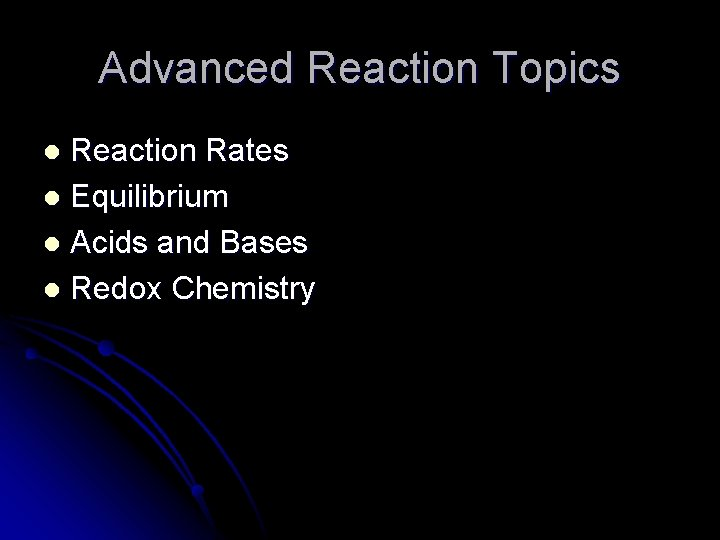Advanced Reaction Topics Reaction Rates l Equilibrium l Acids and Bases l Redox Chemistry lReaction Rates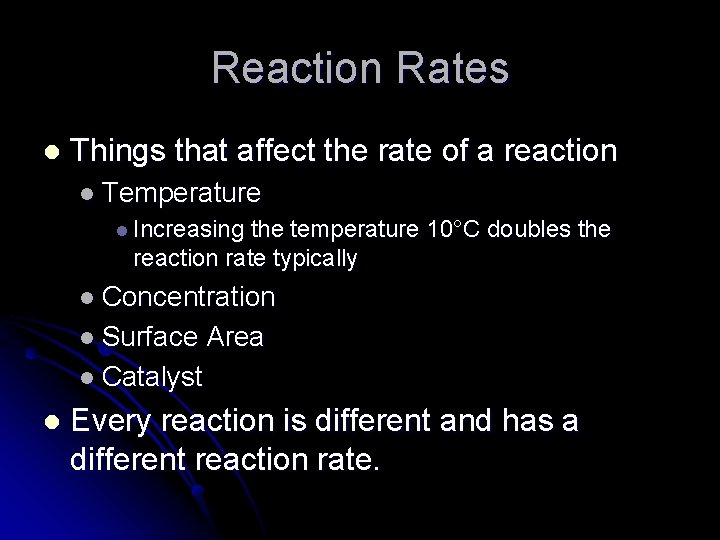Reaction Rates l Things that affect the rate of a reaction l Temperature l Increasing the temperature 10°C doubles the reaction rate typically l Concentration l Surface Area l Catalyst l Every reaction is different and has a different reaction rate.Collision Theory l l Reactions happen when molecules collide with each other The reaction only happens IF: l l They collide with enough energy They collide with the right orientation.How Do They Relate? l Things that affect the rate of a reaction l Temperature l Increasing Temperature increases the energy in a collision. l Concentration l Increases the number of particles in the same volume and therefore the number of collisions l Surface Area l Increases the accessible area for collisions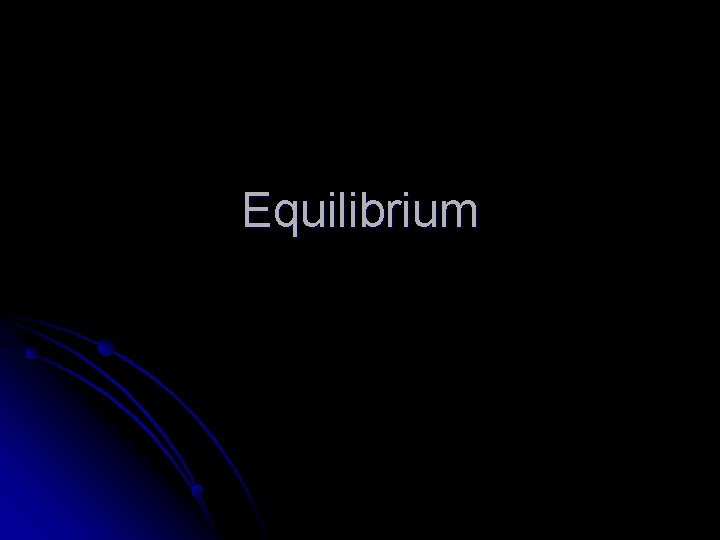EquilibriumFishbowl Demo l What are the characteristics once it reaches equilibrium? l The amount of “reactants” and “products” is not changing l “Reactions” are still occurring in both the forward and reverse direction. l The rate of the “reactions” is the same at equilibrium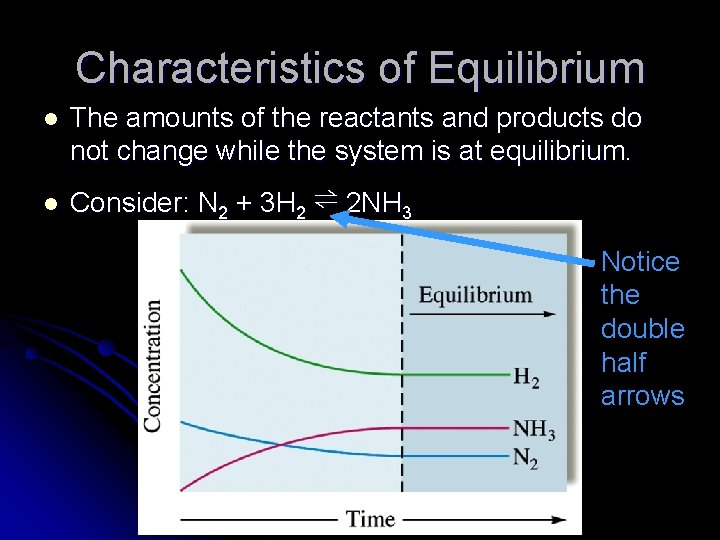Characteristics of Equilibrium l l The amounts of the reactants and products do not change while the system is at equilibrium. Consider: N 2 + 3 H 2 ⇌ 2 NH 3 Notice the double half arrows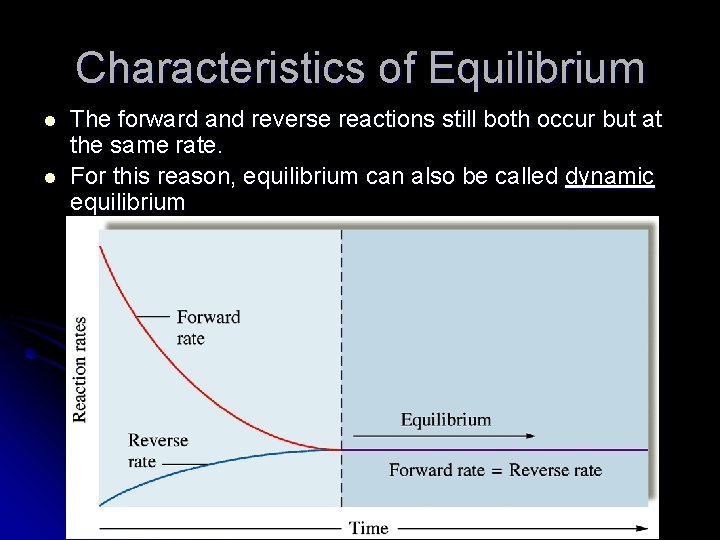Characteristics of Equilibrium l l The forward and reverse reactions still both occur but at the same rate. For this reason, equilibrium can also be called dynamic equilibrium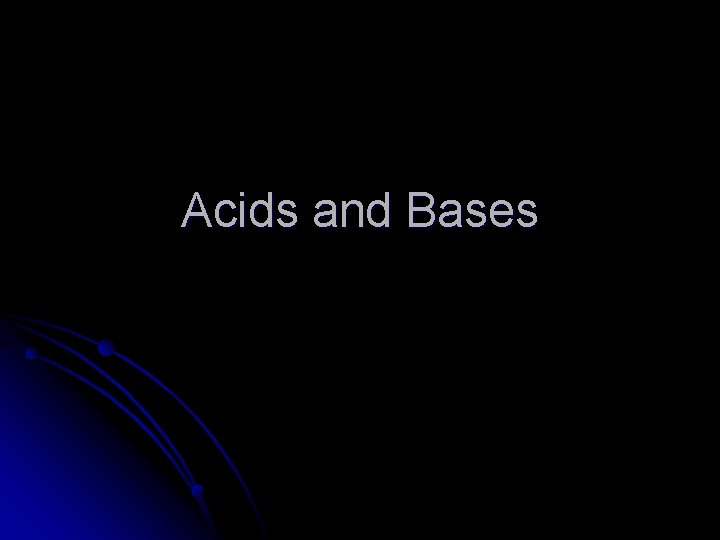Acids and BasesHydrochloric Acid l HCl(aq) l What type of compound is it? l What type of electrolyte is hydrochloric acid?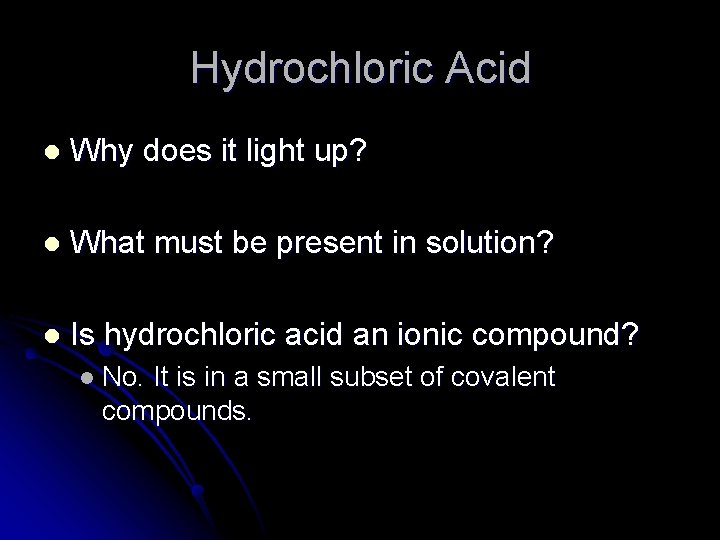Hydrochloric Acid l Why does it light up? l What must be present in solution? l Is hydrochloric acid an ionic compound? l No. It is in a small subset of covalent compounds.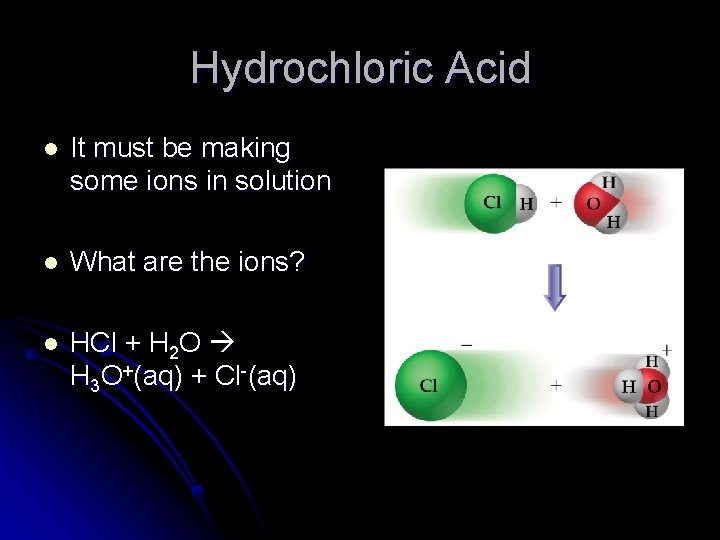Hydrochloric Acid l It must be making some ions in solution l What are the ions? l HCl + H 2 O H 3 O+(aq) + Cl-(aq)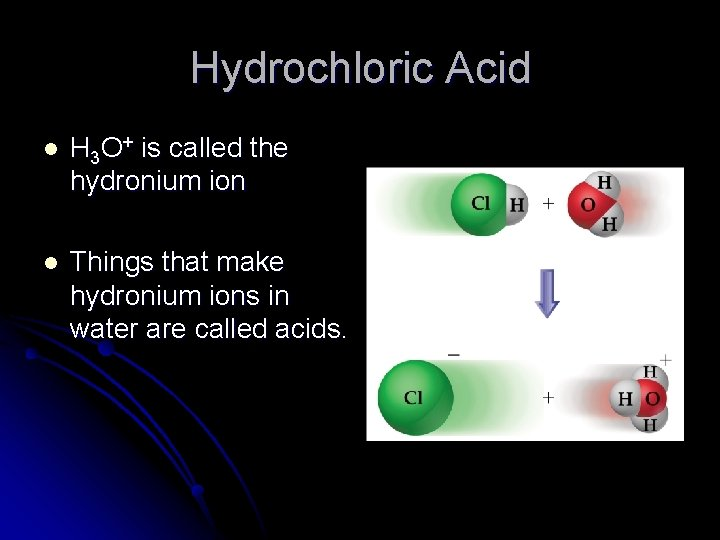Hydrochloric Acid l H 3 O+ is called the hydronium ion l Things that make hydronium ions in water are called acids.A Word About Hydronium l Depending on how you look at it acids make l H+ - hydrogen ion l H 3 O+ - hydronium ion l The two are interchangable l H+ + l H 2 O H 3 O + Anytime you see one it can mean the other.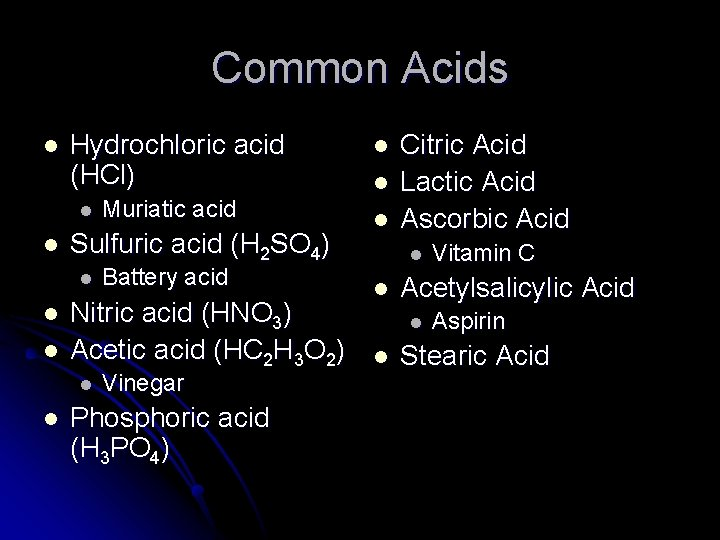Common Acids l Hydrochloric acid (HCl) l l Sulfuric acid (H 2 SO 4) l l l Battery acid Nitric acid (HNO 3) Acetic acid (HC 2 H 3 O 2) l l Muriatic acid Vinegar Phosphoric acid (H 3 PO 4) l l l Citric Acid Lactic Acid Ascorbic Acid l l Acetylsalicylic Acid l l Vitamin C Aspirin Stearic Acid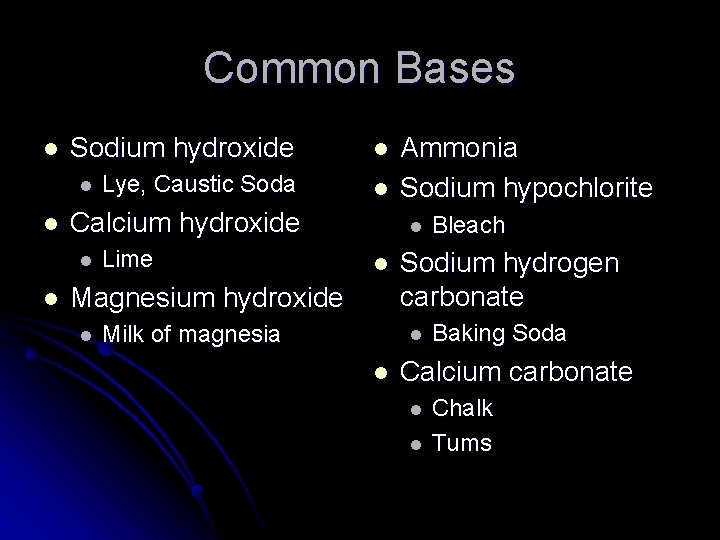Common Bases l Sodium hydroxide l Lye, Caustic Soda l l l Calcium hydroxide l l Lime l l Magnesium hydroxide l Ammonia Sodium hypochlorite Milk of magnesia Sodium hydrogen carbonate l l Bleach Baking Soda Calcium carbonate l l Chalk Tums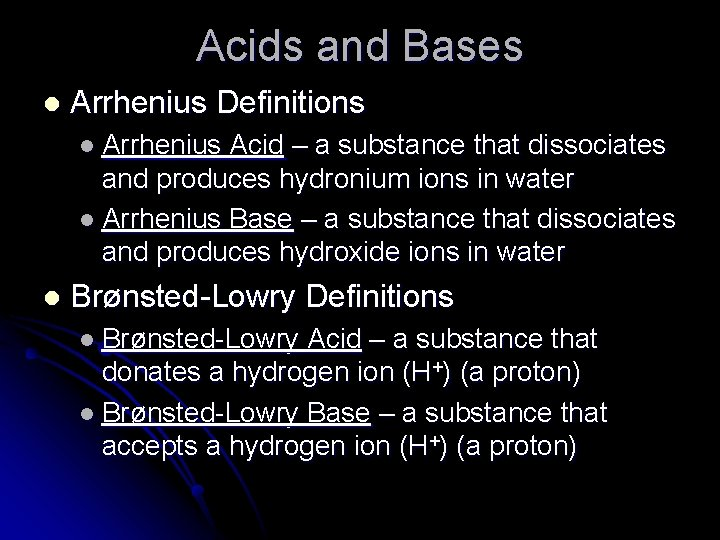Acids and Bases l Arrhenius Definitions l Arrhenius Acid – a substance that dissociates and produces hydronium ions in water l Arrhenius Base – a substance that dissociates and produces hydroxide ions in water l Brønsted-Lowry Definitions l Brønsted-Lowry Acid – a substance that donates a hydrogen ion (H+) (a proton) l Brønsted-Lowry Base – a substance that accepts a hydrogen ion (H+) (a proton)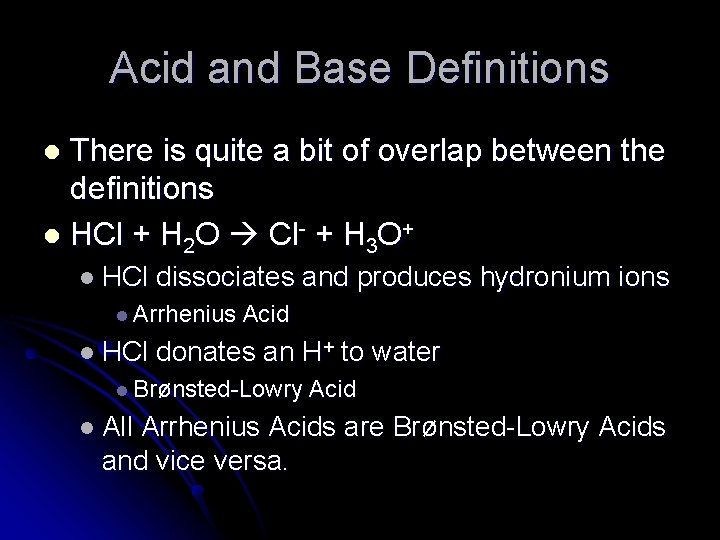Acid and Base Definitions There is quite a bit of overlap between the definitions l HCl + H 2 O Cl- + H 3 O+ l l HCl dissociates and produces hydronium ions l Arrhenius l HCl Acid donates an H+ to water l Brønsted-Lowry l All Acid Arrhenius Acids are Brønsted-Lowry Acids and vice versa.Acid and Base Definitions l Relations between the base definitions are not as simple. l Na. OH dissolves in water to form Na+ and OHl l Na. OH + H+ Na+ + H 2 O l l l Brønsted-Lowry Base Hydroxides are both Arrhenius and Brønsted-Lowry Bases. Non-hydroxide bases can’t be Arrhenius bases l l Arrhenius Base No hydroxide obviously… Non-hydroxide bases will be Brønsted-Lowry bases l l CO 32 - + H+ HCO 3 NH 3 + H+ NH 4+Acid and Base Definitions Arrhenius acids and bases are tied to water l Brønsted-Lowry acids and bases are not. l l Brønsted-Lowry can be used to describe reactions in the gas phase or in other solvents besides water.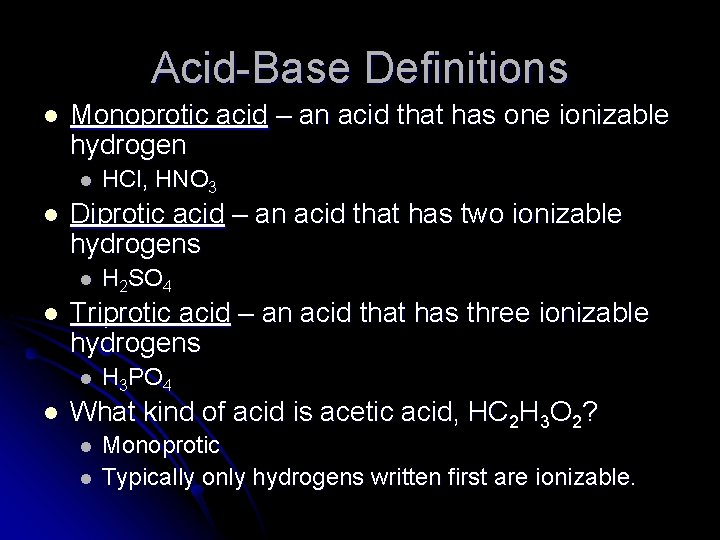Acid-Base Definitions l Monoprotic acid – an acid that has one ionizable hydrogen l l Diprotic acid – an acid that has two ionizable hydrogens l l H 2 SO 4 Triprotic acid – an acid that has three ionizable hydrogens l l HCl, HNO 3 H 3 PO 4 What kind of acid is acetic acid, HC 2 H 3 O 2? l l Monoprotic Typically only hydrogens written first are ionizable.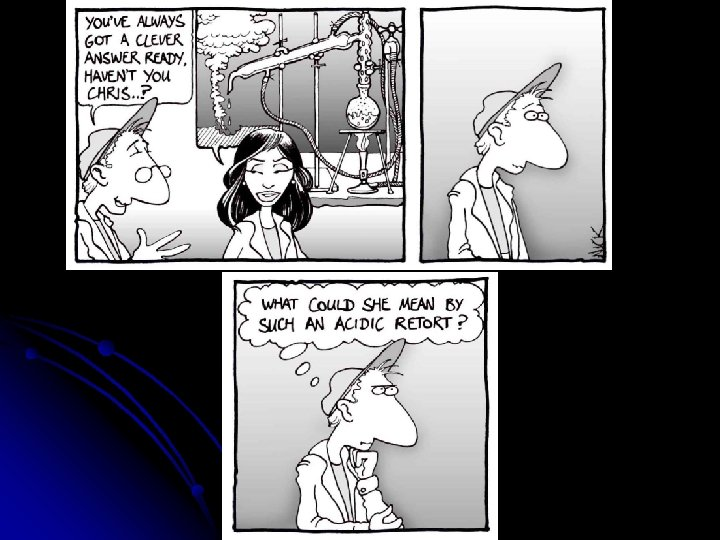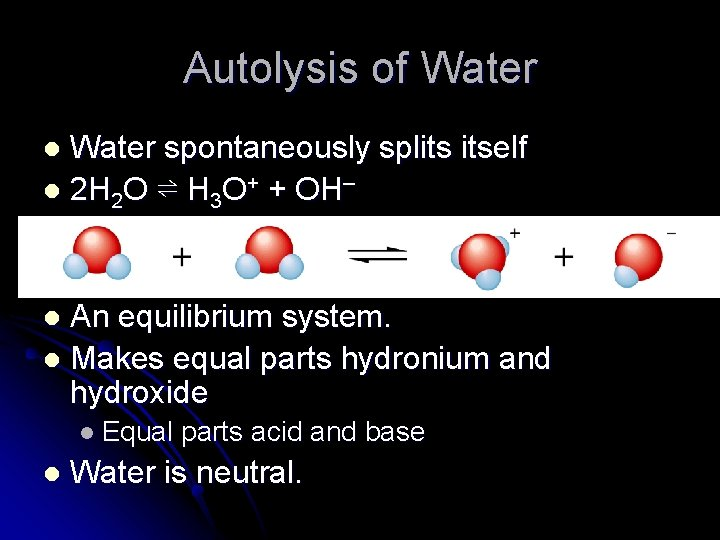Autolysis of Water spontaneously splits itself l 2 H 2 O ⇌ H 3 O+ + OH– l An equilibrium system. l Makes equal parts hydronium and hydroxide l l Equal l parts acid and base Water is neutral.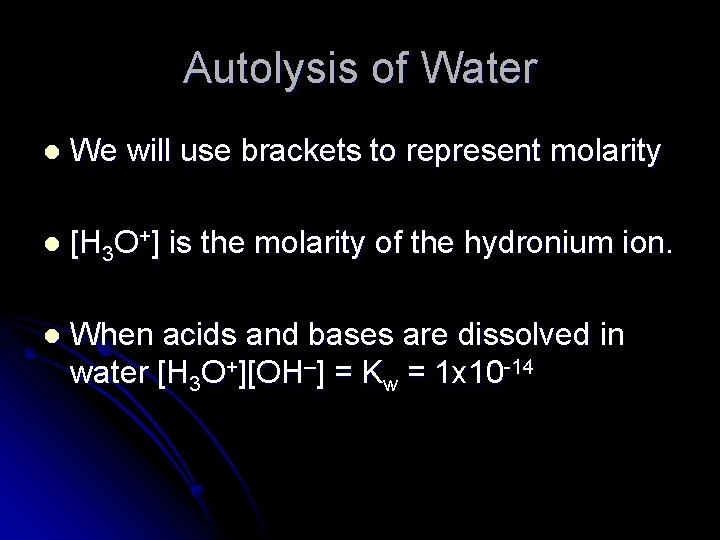Autolysis of Water l We will use brackets to represent molarity l [H 3 O+] is the molarity of the hydronium ion. l When acids and bases are dissolved in water [H 3 O+][OH–] = Kw = 1 x 10 -14Acid Base Reactions l Acid Base Neutralization Reaction l HA + B A + HB l Transfer of hydrogen ions (H+) l Hydrochloric acid and sodium hydroxide solutions are mixed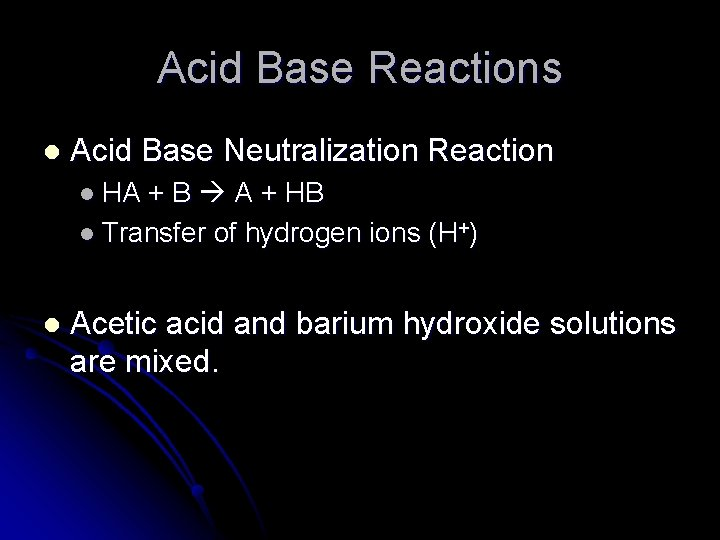Acid Base Reactions l Acid Base Neutralization Reaction l HA + B A + HB l Transfer of hydrogen ions (H+) l Acetic acid and barium hydroxide solutions are mixed.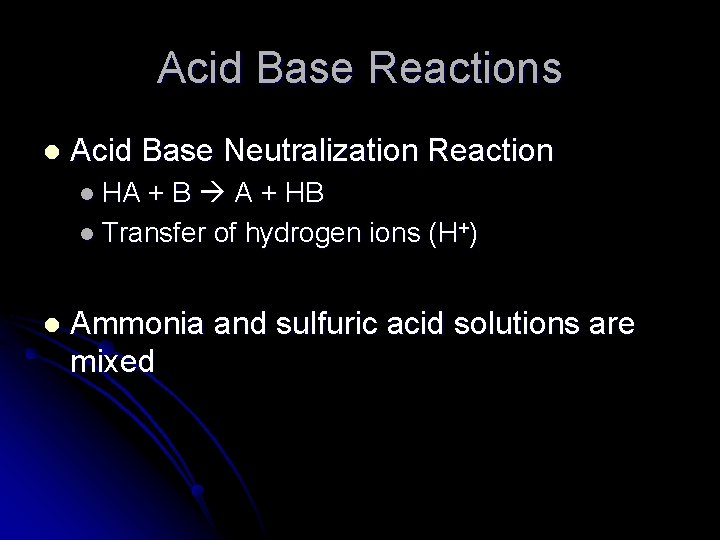Acid Base Reactions l Acid Base Neutralization Reaction l HA + B A + HB l Transfer of hydrogen ions (H+) l Ammonia and sulfuric acid solutions are mixedAcid Base Reactions l Acid Base Neutralization Reaction l HA + B A + HB l Transfer of hydrogen ions (H+) l Hydrochloric acid and sodium sulfide solutions are mixed.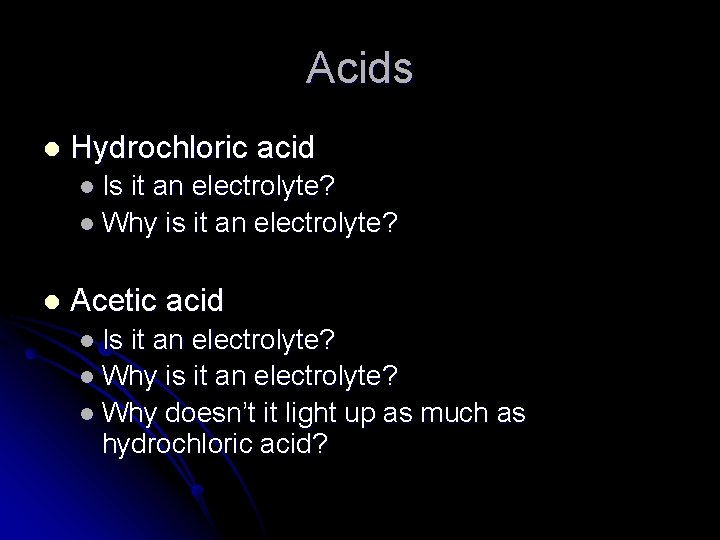Acids l Hydrochloric acid l Is it an electrolyte? l Why is it an electrolyte? l Acetic acid l Is it an electrolyte? l Why is it an electrolyte? l Why doesn’t it light up as much as hydrochloric acid?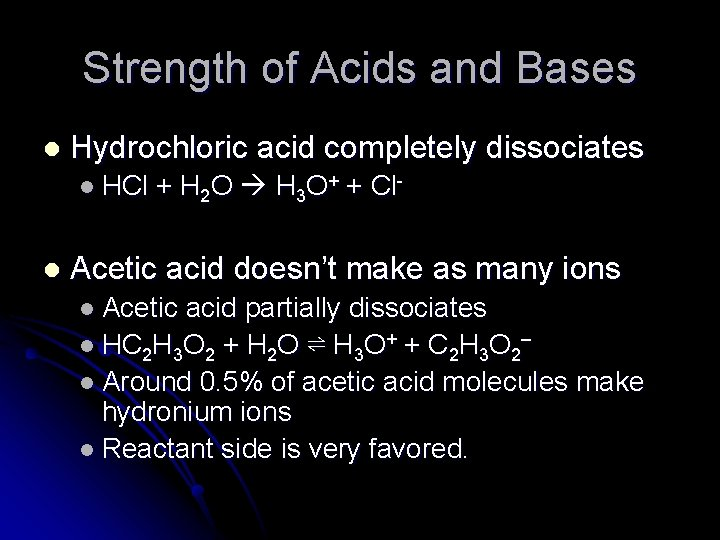Strength of Acids and Bases l Hydrochloric acid completely dissociates l HCl l + H 2 O H 3 O+ + Cl- Acetic acid doesn’t make as many ions l Acetic acid partially dissociates l HC 2 H 3 O 2 + H 2 O ⇌ H 3 O+ + C 2 H 3 O 2– l Around 0. 5% of acetic acid molecules make hydronium ions l Reactant side is very favored.Strength of Acids and Bases l Strong acid or base – an acid or base that completely reacts with water to form hydronium ions or hydroxide ions. l l l Strong acids – hydrochloric, sulfuric, nitric Strong bases – Li. OH, Na. OH, KOH, Rb. OH, Cs. OH, Ca(OH)2, Sr(OH)2, Ba(OH)2 Weak acid or base – an acid or base that partially reacts with water to form hydronium ions or hydroxide ions. l l Weak acids – everything except the above Weak bases – everything except the above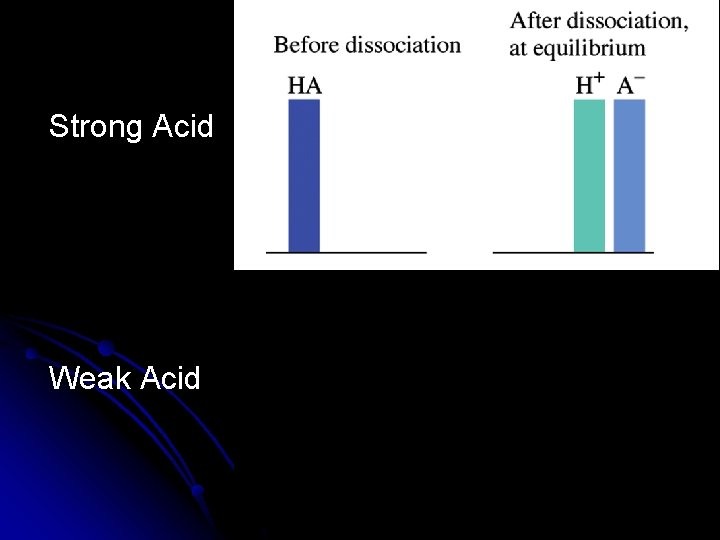Strong Acid Weak Acid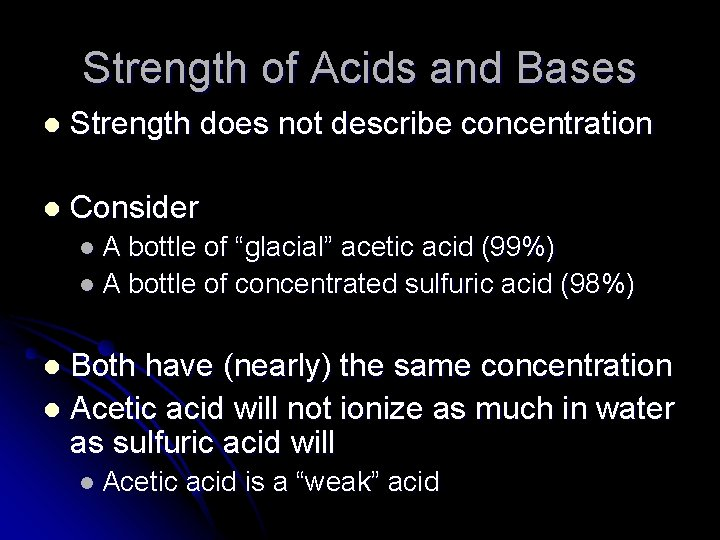Strength of Acids and Bases l Strength does not describe concentration l Consider l. A bottle of “glacial” acetic acid (99%) l A bottle of concentrated sulfuric acid (98%) Both have (nearly) the same concentration l Acetic acid will not ionize as much in water as sulfuric acid will l l Acetic acid is a “weak” acidConcentration of Acids l If not all acids completely dissociate in water l The concentration of the acid molecules is different for every acid l The concentration of the hydronium ion in different acids is different l Need a system that describes the concentration of acids.p. H l p. H = -log [H 3 O+] Also define a relationship for the amount of base l p. OH = -log [OH–] l How do you reverse a log? l 10 -p. H = [H 3 O+] l 10 -p. OH = [OH–] l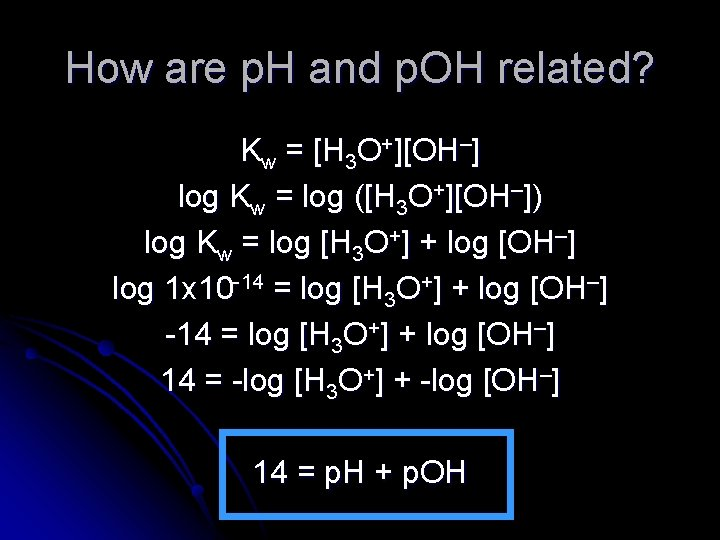How are p. H and p. OH related? Kw = [H 3 O+][OH–] log Kw = log ([H 3 O+][OH–]) log Kw = log [H 3 O+] + log [OH–] log 1 x 10 -14 = log [H 3 O+] + log [OH–] 14 = -log [H 3 O+] + -log [OH–] 14 = p. H + p. OH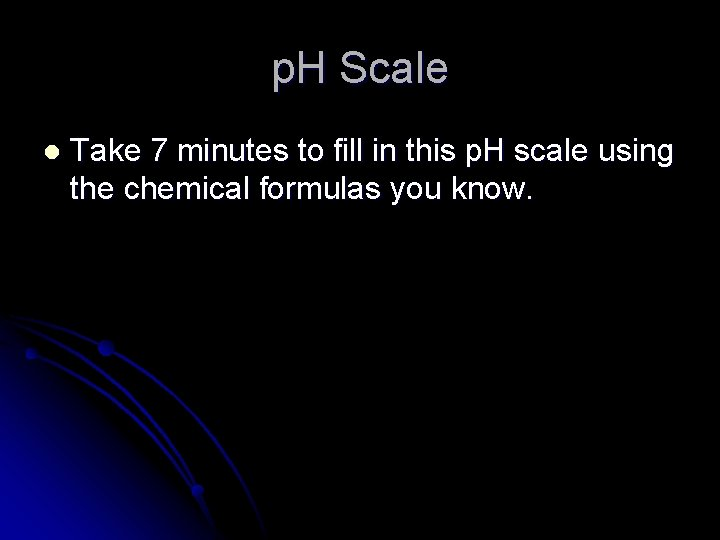p. H Scale l Take 7 minutes to fill in this p. H scale using the chemical formulas you know.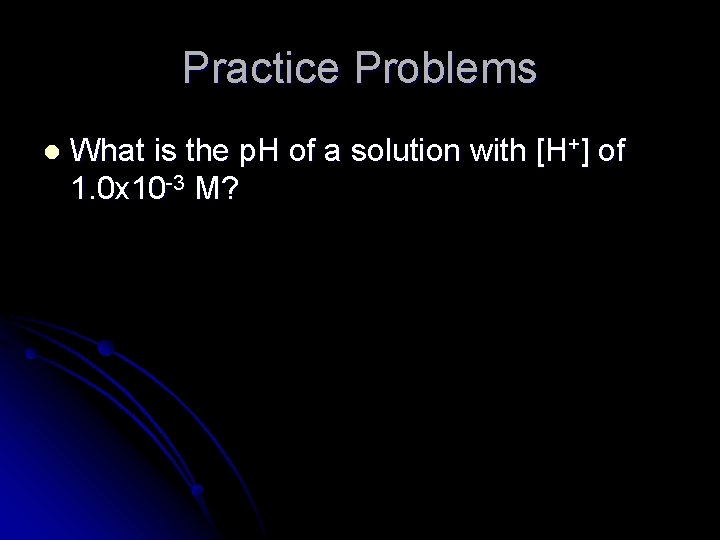Practice Problems l What is the p. H of a solution with [H+] of 1. 0 x 10 -3 M?Practice Problems l What is the p. OH of a solution with [H+] of 1. 0 x 10 -3 M?Practice Problems l What is the [OH-] of a solution with a p. H of 9. 00?Practice Problems l What is the [OH-] of a solution with an [H 3 O+] concentration of 1. 0 x 10 -5 M?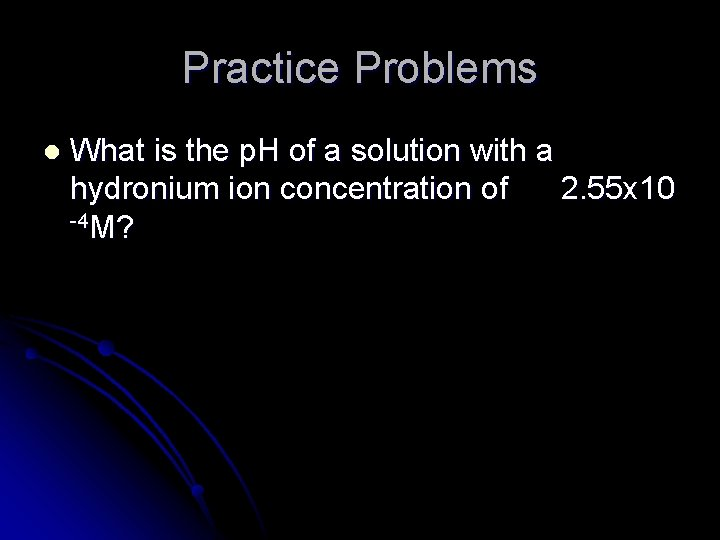Practice Problems l What is the p. H of a solution with a hydronium ion concentration of 2. 55 x 10 -4 M?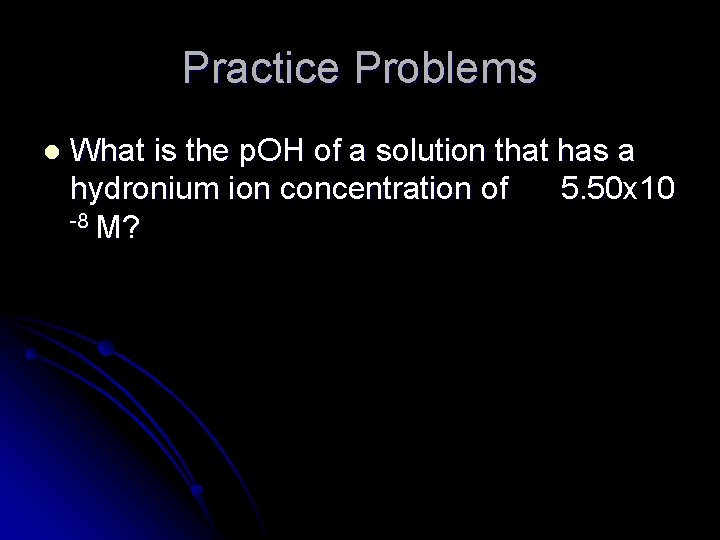Practice Problems l What is the p. OH of a solution that has a hydronium ion concentration of 5. 50 x 10 -8 M?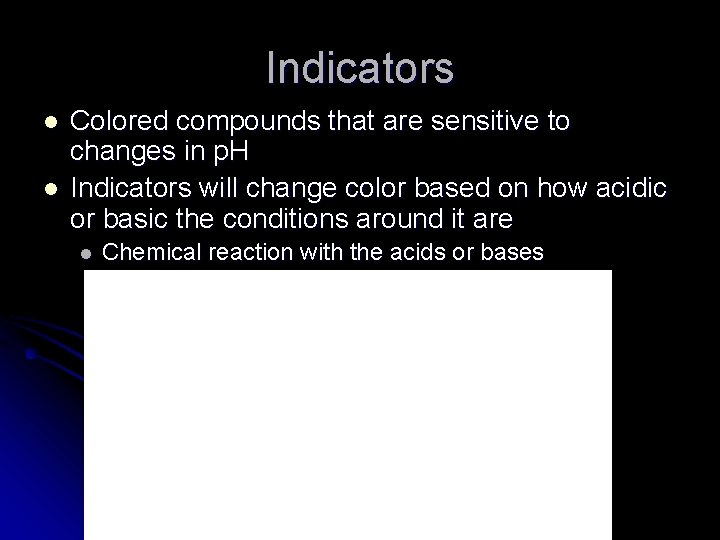Indicators l l Colored compounds that are sensitive to changes in p. H Indicators will change color based on how acidic or basic the conditions around it are l Chemical reaction with the acids or basesUniversal Indicator l Universal Indicator is a mixture of different indicators. l Resulting color depends on each of the four indicator’s states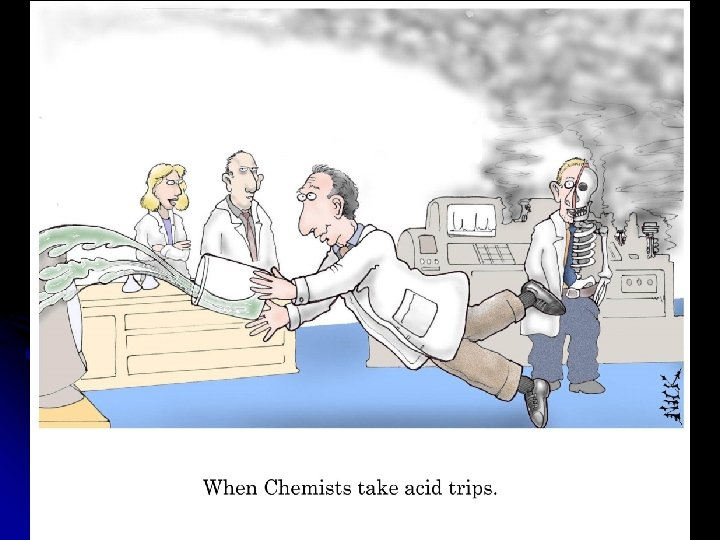Redox Chemistry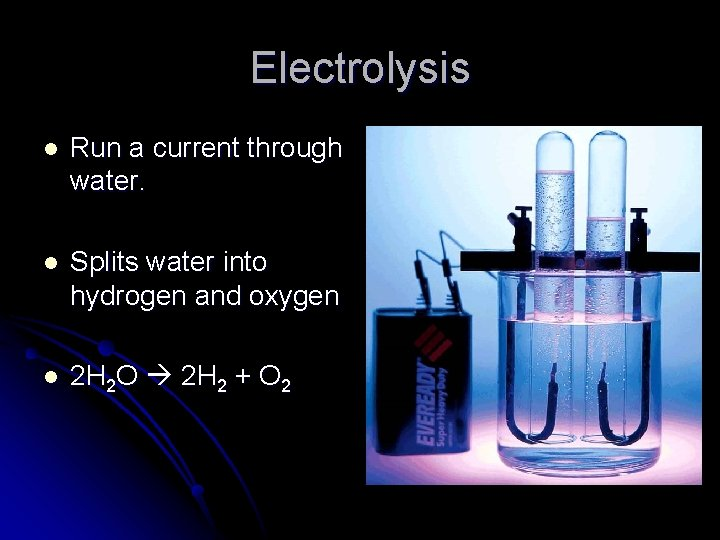Electrolysis l Run a current through water. l Splits water into hydrogen and oxygen l 2 H 2 O 2 H 2 + O 2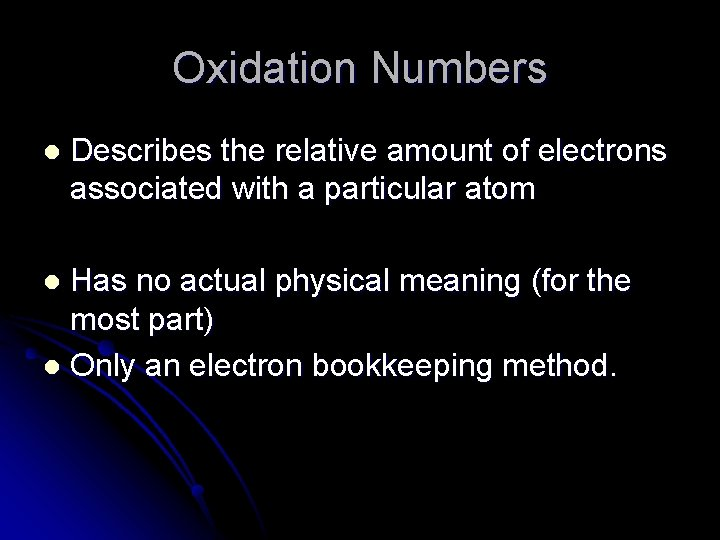Oxidation Numbers l Describes the relative amount of electrons associated with a particular atom Has no actual physical meaning (for the most part) l Only an electron bookkeeping method. l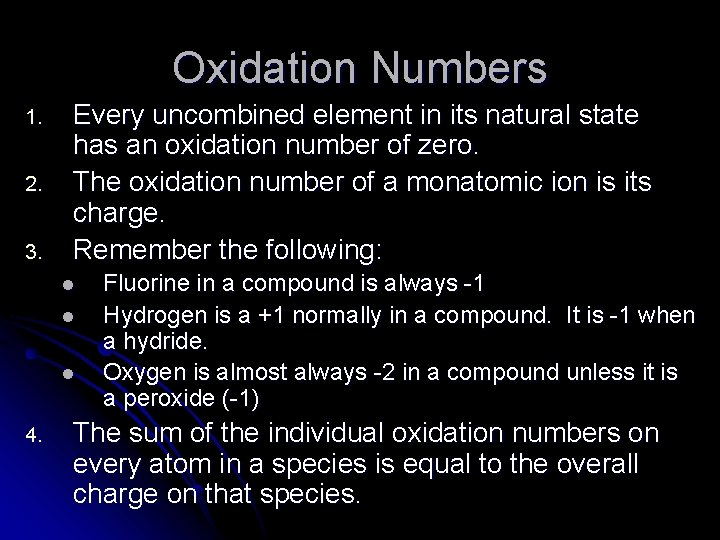Oxidation Numbers 1. 2. 3. Every uncombined element in its natural state has an oxidation number of zero. The oxidation number of a monatomic ion is its charge. Remember the following: l l l 4. Fluorine in a compound is always -1 Hydrogen is a +1 normally in a compound. It is -1 when a hydride. Oxygen is almost always -2 in a compound unless it is a peroxide (-1) The sum of the individual oxidation numbers on every atom in a species is equal to the overall charge on that species.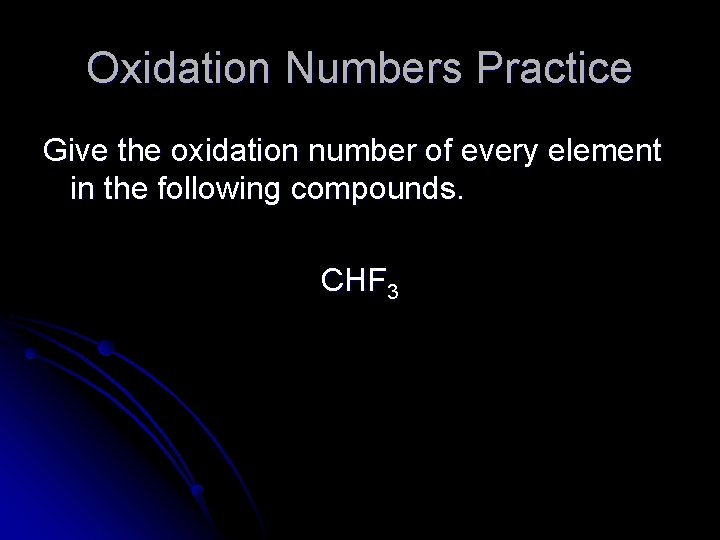Oxidation Numbers Practice Give the oxidation number of every element in the following compounds. CHF 3Oxidation Numbers Practice Give the oxidation number of every element in the following compounds. Ba. Cl 2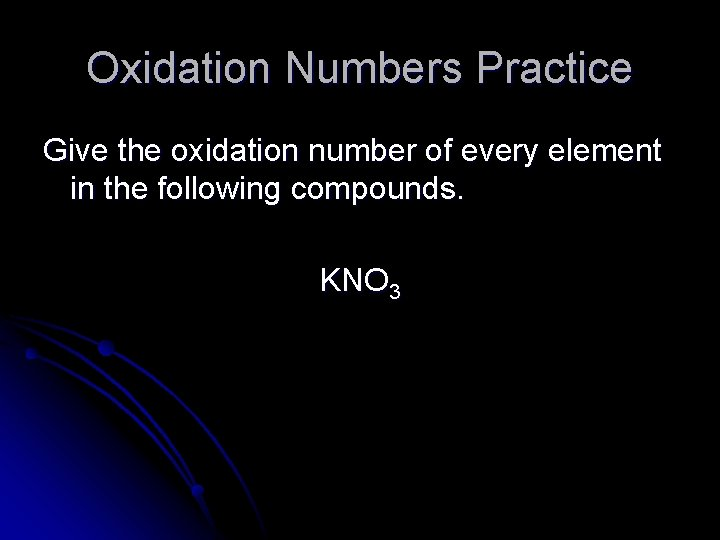Oxidation Numbers Practice Give the oxidation number of every element in the following compounds. KNO 3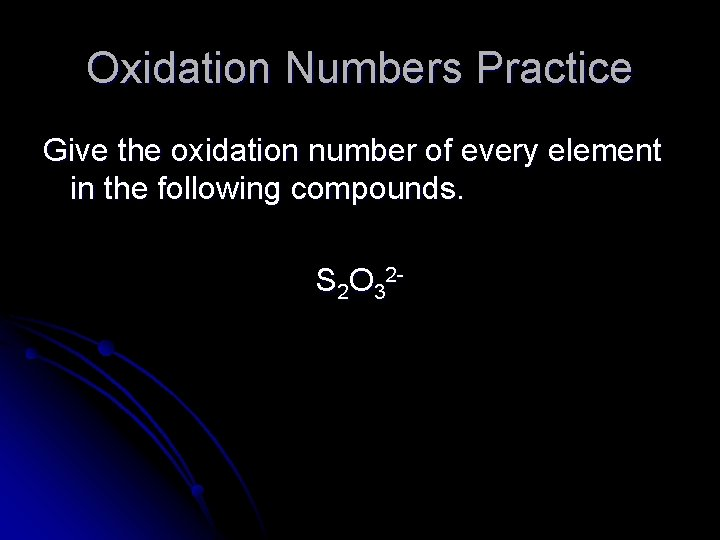Oxidation Numbers Practice Give the oxidation number of every element in the following compounds. S 2 O 32 -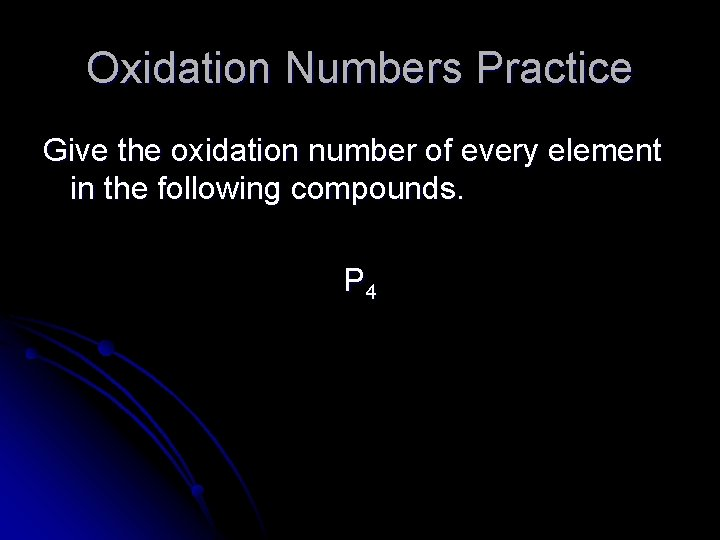Oxidation Numbers Practice Give the oxidation number of every element in the following compounds. P 4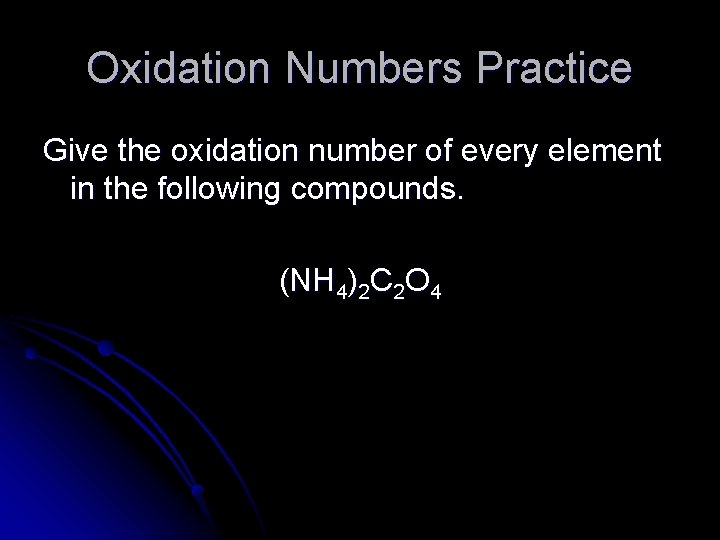Oxidation Numbers Practice Give the oxidation number of every element in the following compounds. (NH 4)2 C 2 O 4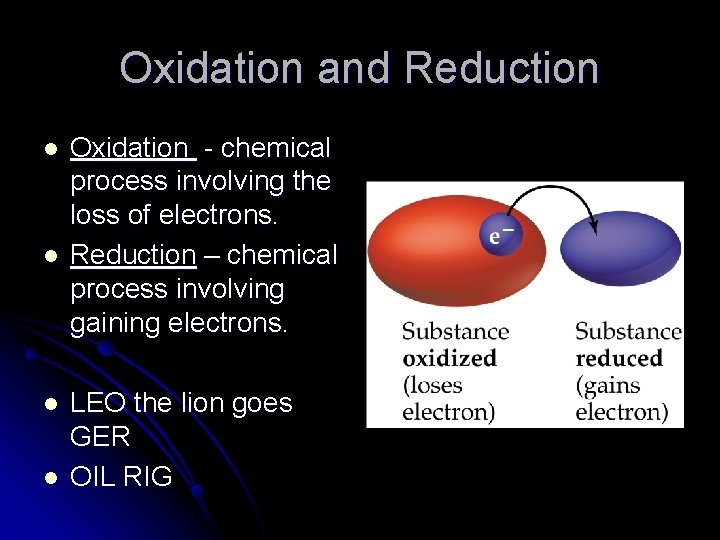Oxidation and Reduction l l Oxidation - chemical process involving the loss of electrons. Reduction – chemical process involving gaining electrons. LEO the lion goes GER OIL RIGOxidation and Reduction l Consider: Mn. O 4 - + C 2 O 42 - + H+ Mn 2+ + CO 2 + H 2 O l l l What element has been reduced? What element has been oxidized? What species reacted with the element that was reduced? l l This is the reducing agent. What species reacted with the element that was oxidized? l This is the oxidizing agent.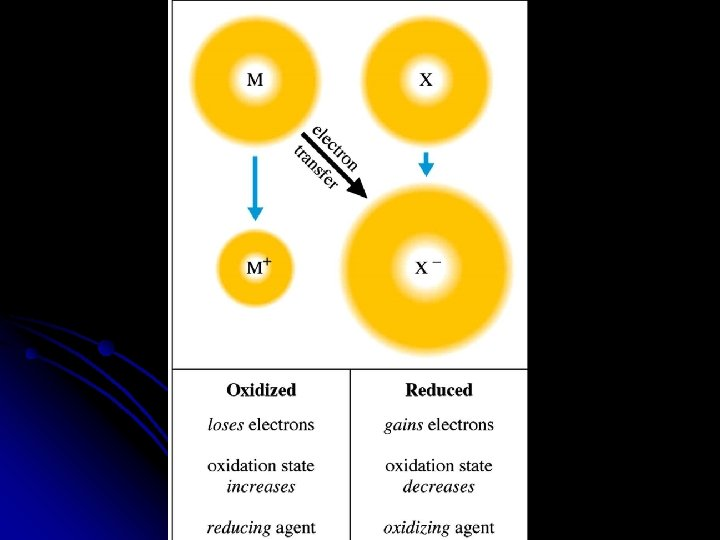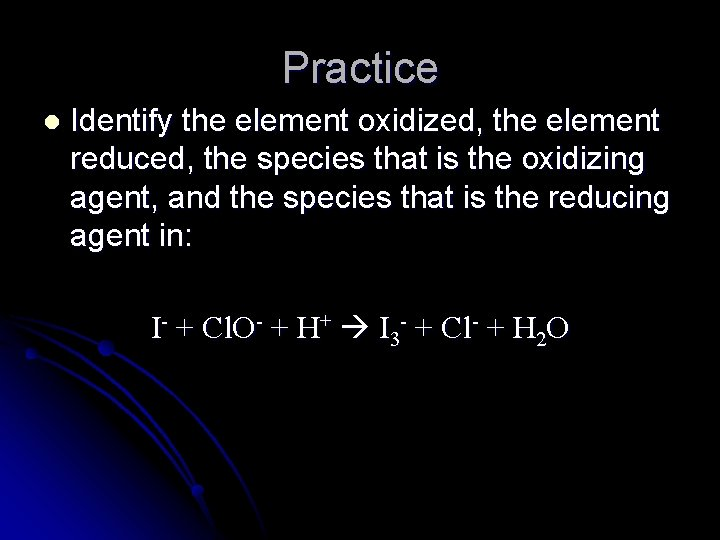Practice l Identify the element oxidized, the element reduced, the species that is the oxidizing agent, and the species that is the reducing agent in: I- + Cl. O- + H+ I 3 - + Cl- + H 2 O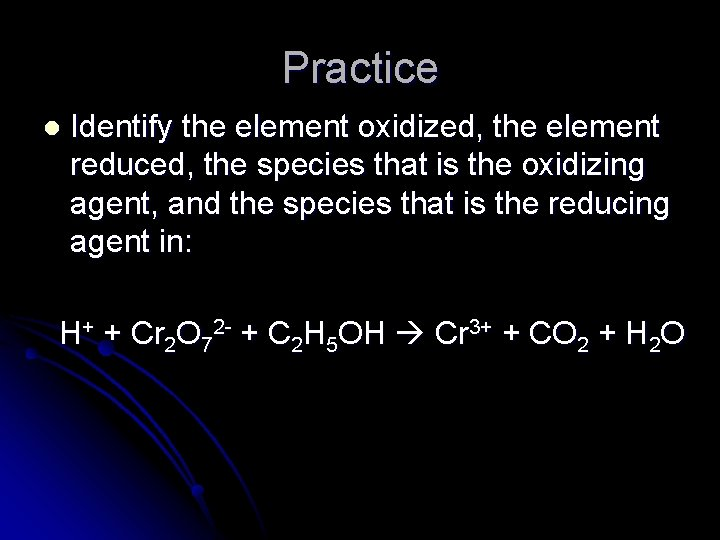Practice l Identify the element oxidized, the element reduced, the species that is the oxidizing agent, and the species that is the reducing agent in: H+ + Cr 2 O 72 - + C 2 H 5 OH Cr 3+ + CO 2 + H 2 O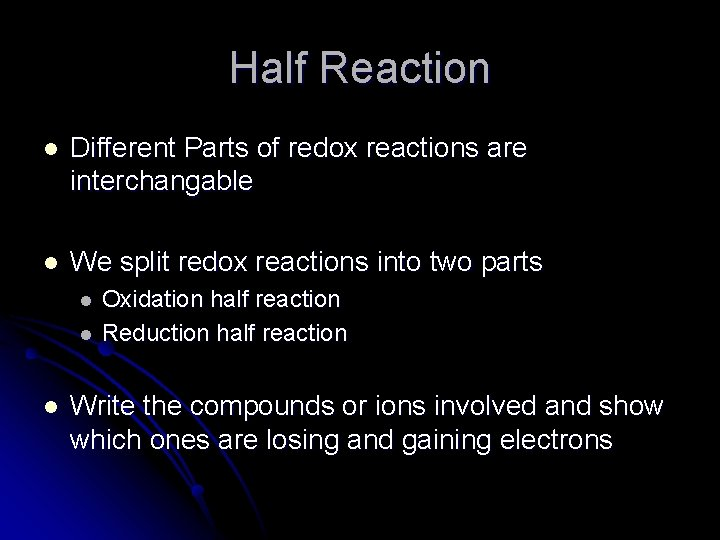Half Reaction l Different Parts of redox reactions are interchangable l We split redox reactions into two parts l l l Oxidation half reaction Reduction half reaction Write the compounds or ions involved and show which ones are losing and gaining electronsPractice Problems Cu + Ag+ Cu 2+ + Ag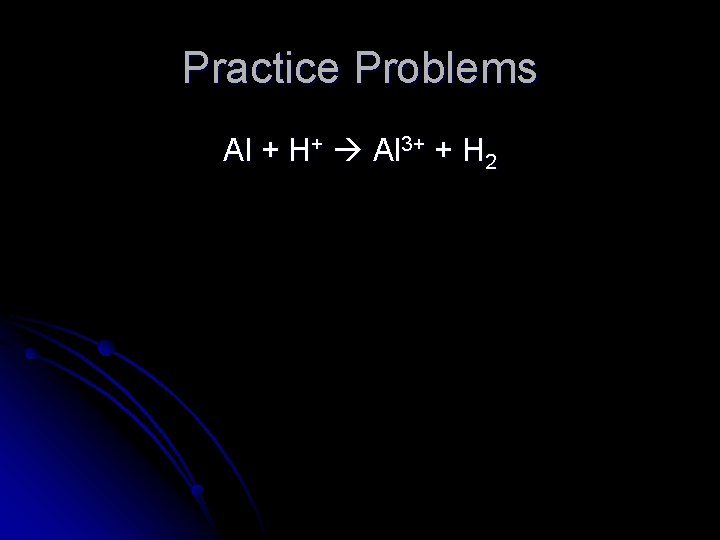Practice Problems Al + H+ Al 3+ + H 2Practice Problems F 2 + Cl- F- + Cl 2Practice Problems Sn 2+ + Fe 3+ Fe 2+ + Sn 4+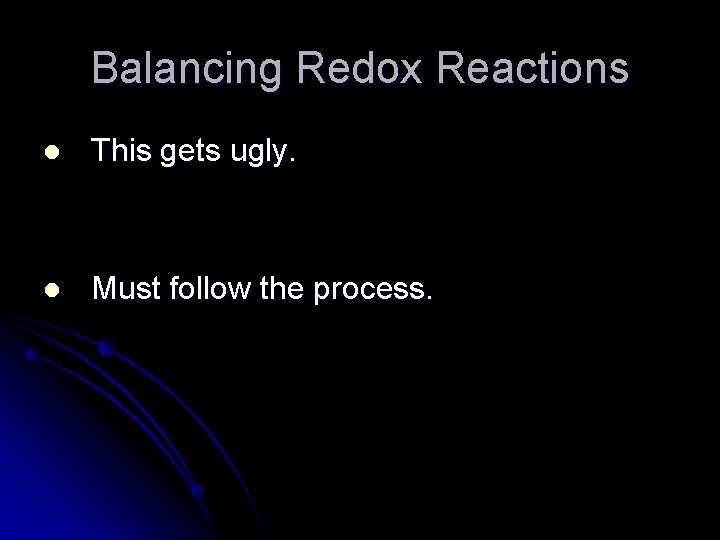Balancing Redox Reactions l This gets ugly. l Must follow the process.The Rules – Copy the Rest 1. 2. Split the overall reaction into two half reactions, one for oxidation and one for reduction. Balance each half reaction separately a) b) c) d) Balance everything but O and H Balance O by adding H 2 O where necessary Balance H by adding H+ where necessary Balance the charge by adding electrons.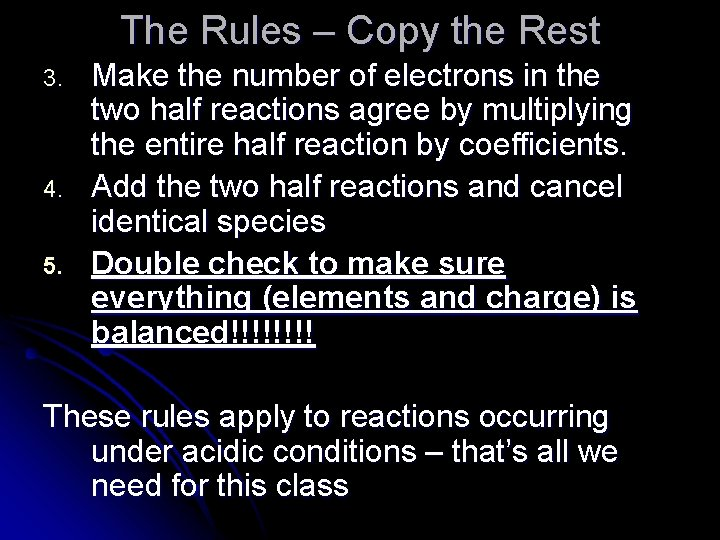The Rules – Copy the Rest 3. 4. 5. Make the number of electrons in the two half reactions agree by multiplying the entire half reaction by coefficients. Add the two half reactions and cancel identical species Double check to make sure everything (elements and charge) is balanced!!!! These rules apply to reactions occurring under acidic conditions – that’s all we need for this class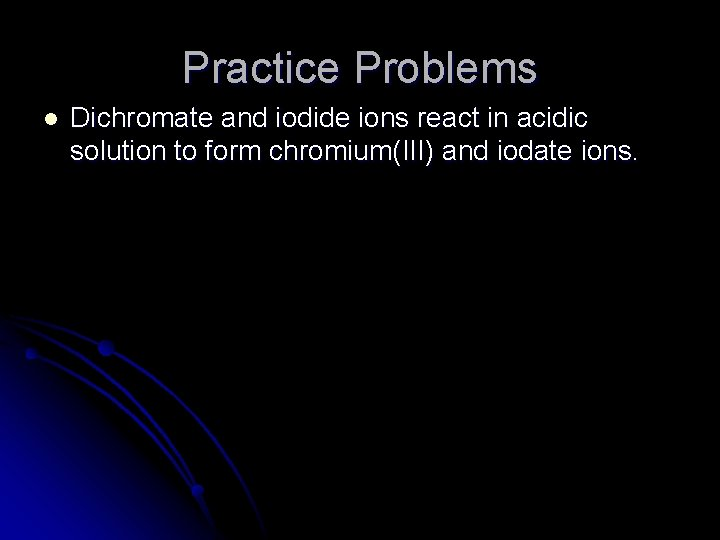Practice Problems l Dichromate and iodide ions react in acidic solution to form chromium(III) and iodate ions.Practice Problems l Permanganate ions and methanol (CH 3 OH) react to form manganese(II) ions and formic acid (HCO 2 H) in acidic solution.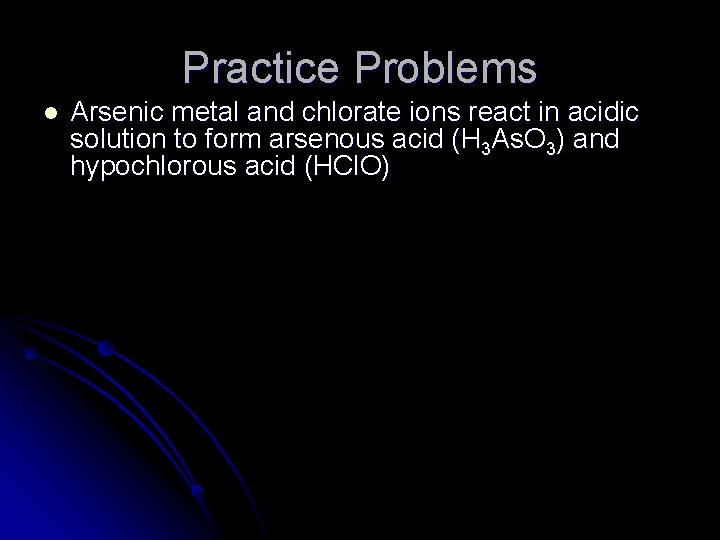Practice Problems l Arsenic metal and chlorate ions react in acidic solution to form arsenous acid (H 3 As. O 3) and hypochlorous acid (HCl. O)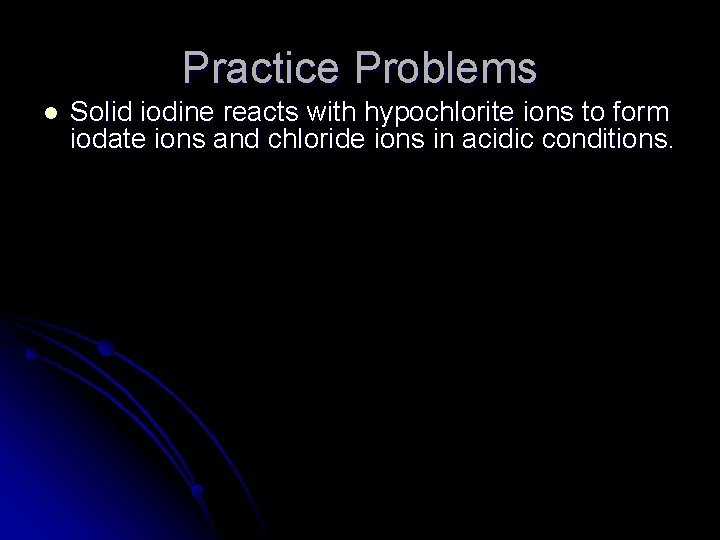Practice Problems l Solid iodine reacts with hypochlorite ions to form iodate ions and chloride ions in acidic conditions.Practice Problems l Arsenic metal and chlorate ions react in acidic solution to form arsenous acid (H 3 As. O 3) and hypochlorous acid (HCl. O) l Solid iodine reacts with hypochlorite ions to form iodate ions and chloride ions in acidic conditions. l Oxalate ions (C 2 O 42 -) and permanganate ions react in acidic conditions to form manganese(II) ions and carbon dioxide. l Dichromate ions react with hydrogen peroxide to produce chromium(III) ions and oxygen gas in acidic conditions l Aluminum metal reacts with iodate ions in acidic solution to form aluminum ions and solid iodine.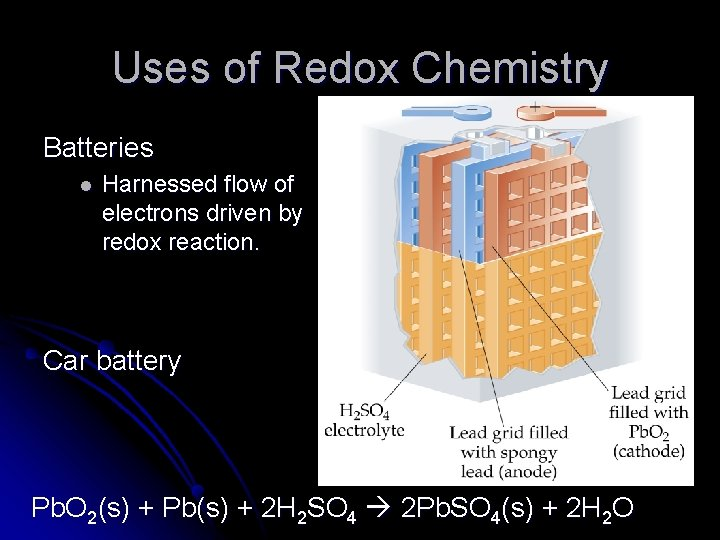Uses of Redox Chemistry Batteries l Harnessed flow of electrons driven by redox reaction. Car battery Pb. O 2(s) + Pb(s) + 2 H 2 SO 4 2 Pb. SO 4(s) + 2 H 2 O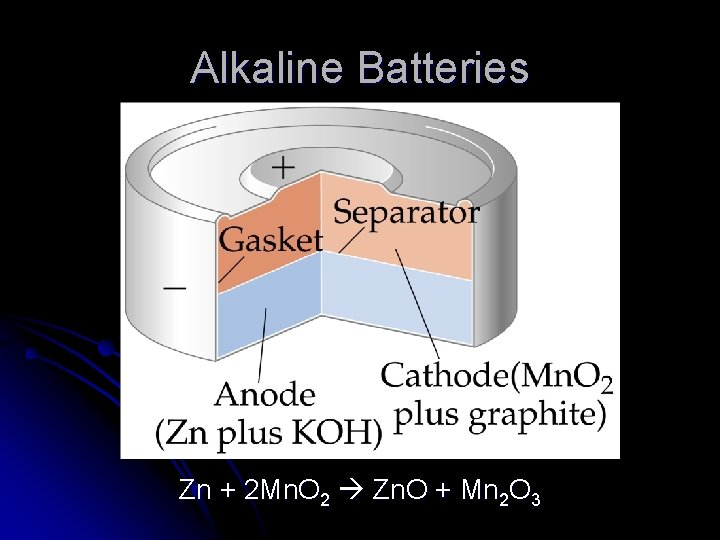Alkaline Batteries Zn + 2 Mn. O 2 Zn. O + Mn 2 O 3Fuel Cells l 2 H 2 + O 2 2 H 2 O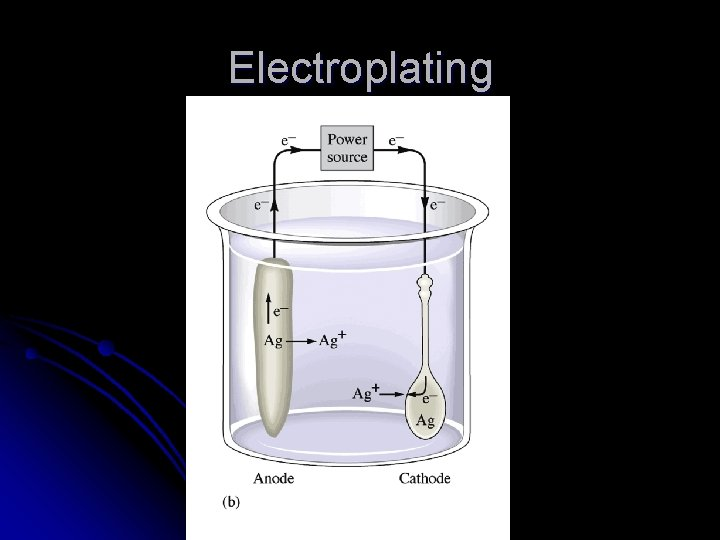ElectroplatingCorrosion